# Unity 宝塔对战教程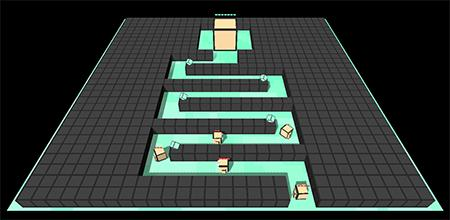Unity 版本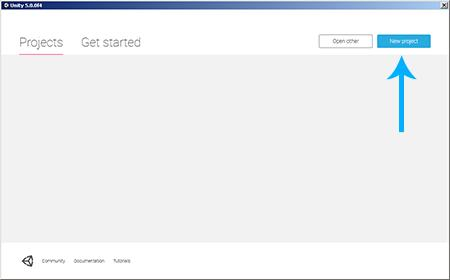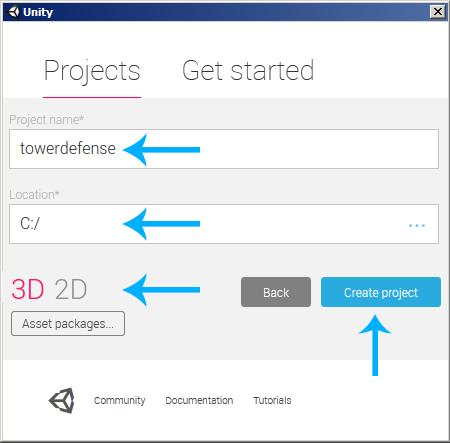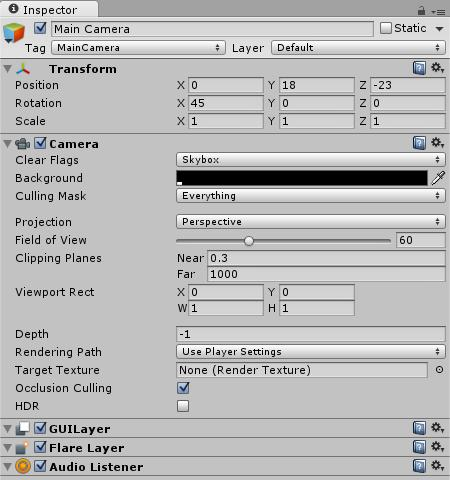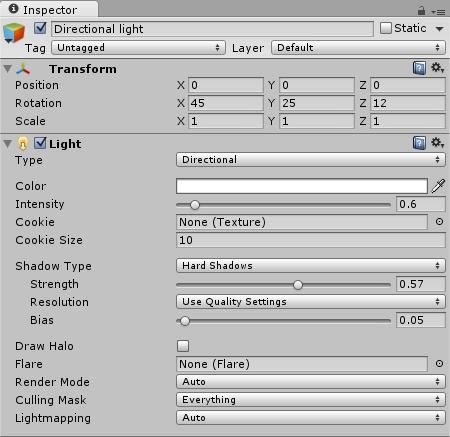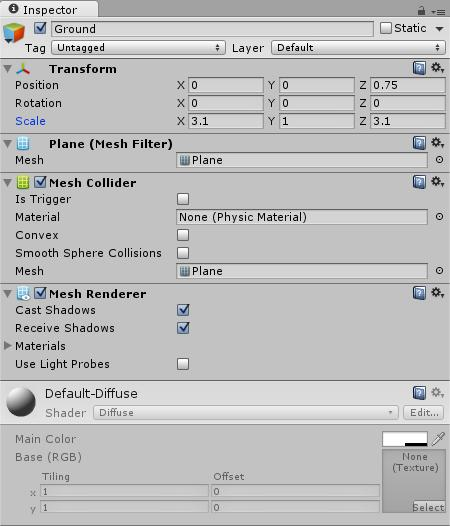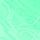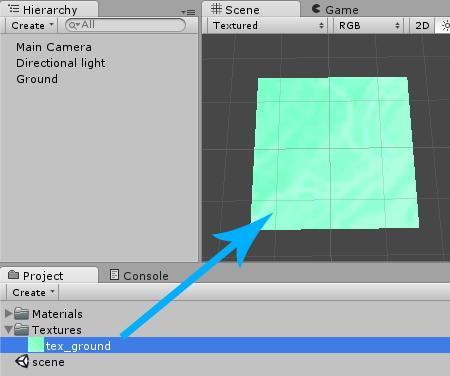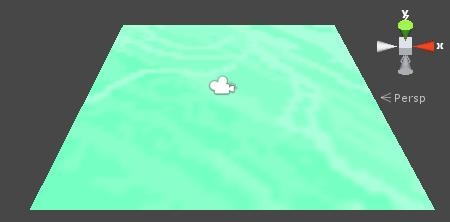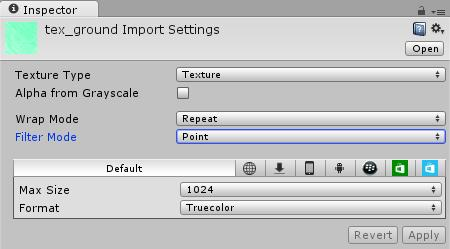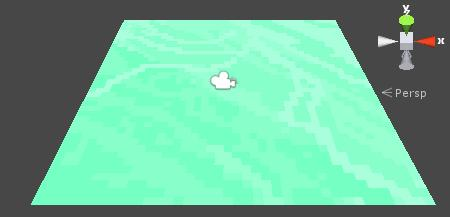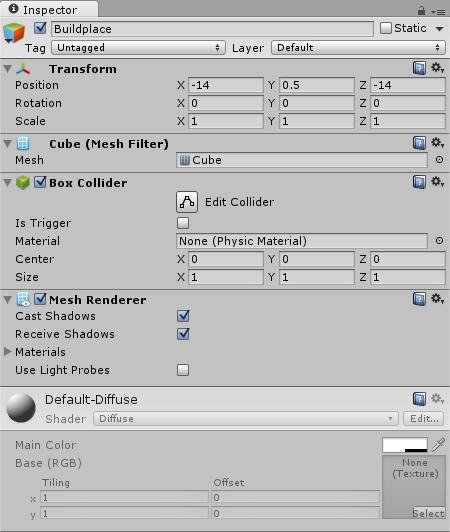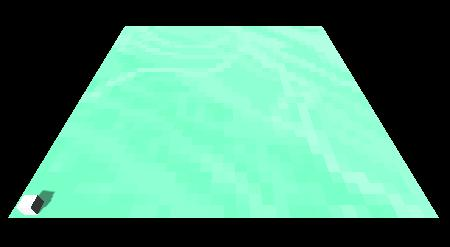Buildplace的纹理

tex_buildplace.png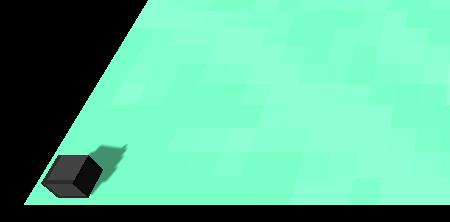Buildplace 脚本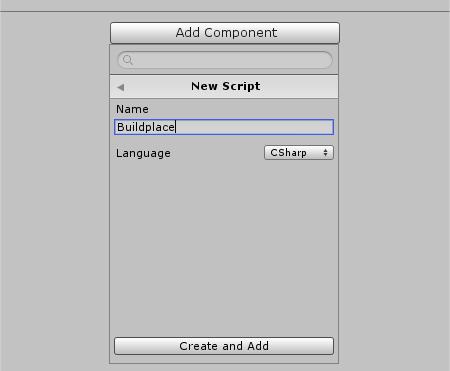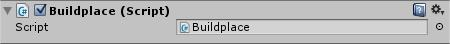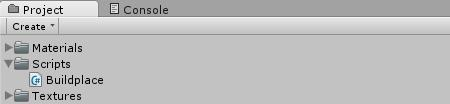[C#] 纯文本查看 复制代码

?

 0102030405060708091011121314 `using` `UnityEngine;``using` `System.Collections;``public` `class` `Buildplace : MonoBehaviour {`` ``// Use this for initialization`` ``void` `Start () {`` ``}`` ``// Update is called once per frame`` ``void` `Update () {`` ``}``}`

[C#] 纯文本查看 复制代码

?

 12345 `using` `UnityEngine;``using` `System.Collections;``public` `class` `Buildplace : MonoBehaviour {``}`

[C#] 纯文本查看 复制代码

?

 1234567 `using` `UnityEngine;``using` `System.Collections;``public` `class` `Buildplace : MonoBehaviour {`` ``// The Tower that should be built`` ``public` `GameObject towerPrefab;``}`

[C#] 纯文本查看 复制代码

?

 01020304050607080910 `using` `UnityEngine;``using` `System.Collections;``public` `class` `Buildplace : MonoBehaviour {`` ``// The Tower that should be built`` ``public` `GameObject towerPrefab;`` ``void` `OnMouseUpAsButton() {`` ``// TODO build stuff...`` ``}``}`

[C#] 纯文本查看 复制代码

?

 1234 `void` `OnMouseUpAsButton() {`` ``// Build Tower above Buildplace`` ``GameObject g = (GameObject)Instantiate(towerPrefab);`` ``g.transform.position = transform.position + Vector3.up;``}`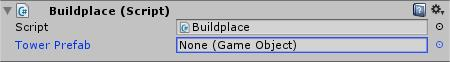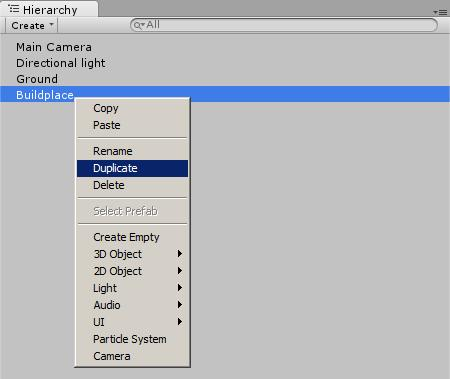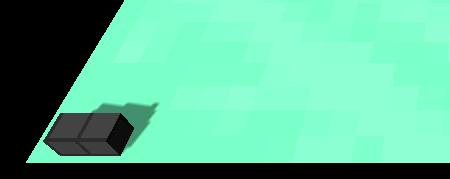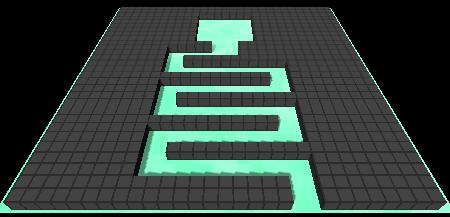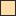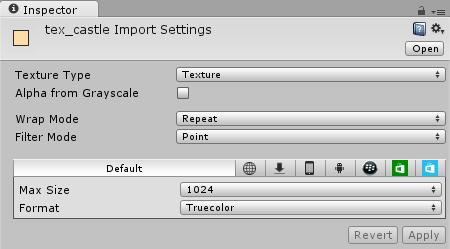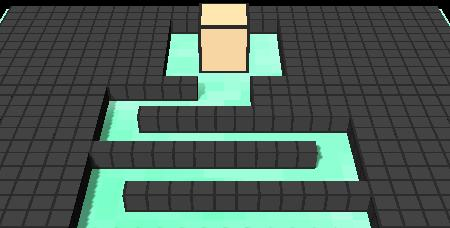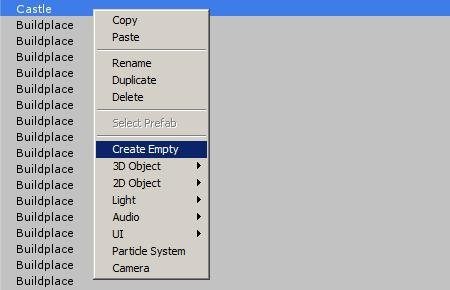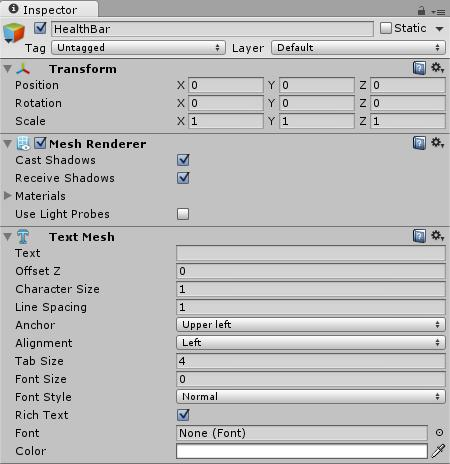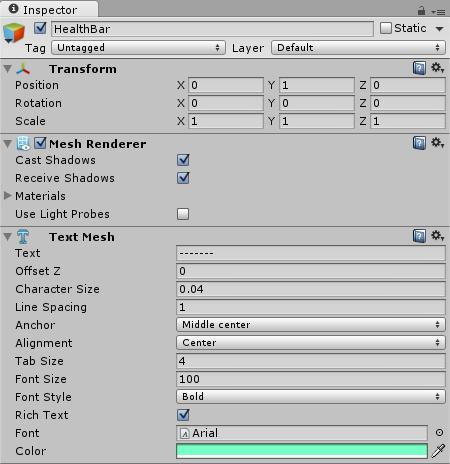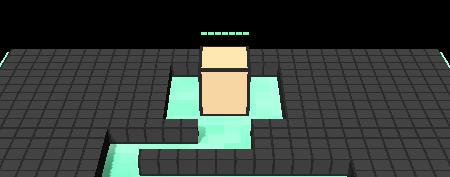[C#] 纯文本查看 复制代码

?

 12345678 `using` `UnityEngine;``using` `System.Collections;``public` `class` `Health : MonoBehaviour {`` ``// Use this for initialization`` ``void` `Start () {`` ``}`

[C#] 纯文本查看 复制代码

?

 1234 ` ``// Update is called once per frame`` ``void` `Update () {`` ``}``}`

[C#] 纯文本查看 复制代码

?

 0102030405060708091011121314 `using` `UnityEngine;``using` `System.Collections;``public` `class` `Health : MonoBehaviour {`` ``// The TextMesh Component`` ``TextMesh tm;`` ``// Use this for initialization`` ``void` `Start () {`` ``tm = GetComponent();`` ``}`` ``// Update is called once per frame`` ``void` `Update () {[/align][align=left]}`

[C#] 纯文本查看 复制代码

?

 0102030405060708091011121314151617 `using` `UnityEngine;``using` `System.Collections;``public` `class` `Health : MonoBehaviour {`` ``// The TextMesh Component`` ``TextMesh tm;`` ``// Use this for initialization`` ``void` `Start () {`` ``tm = GetComponent();`` ``}`` ``// Update is called once per frame`` ``void` `Update () {`` ``// Face the Camera`` ``transform.forward = Camera.main.transform.forward;`` ``}``}`

[C#] 纯文本查看 复制代码

?

 0102030405060708091011 `// Return the current Health by counting the '-'``public` `int` `current() {`` ``return` `tm.text.Length;``}``// Decrease the current Health by removing one '-'``public` `void` `decrease() {`` ``if` `(current() > 1)`` ``tm.text = tm.text.Remove(tm.text.Length - 1);`` ``else`` ``Destroy(transform.parent.gameObject);``}`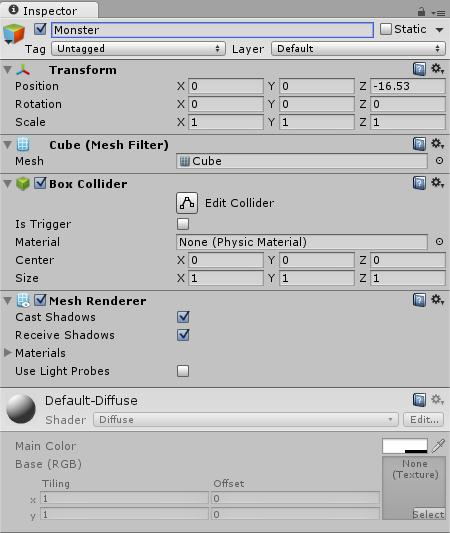tex_monster.png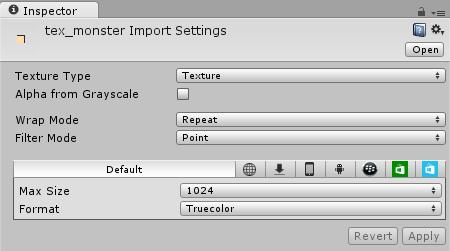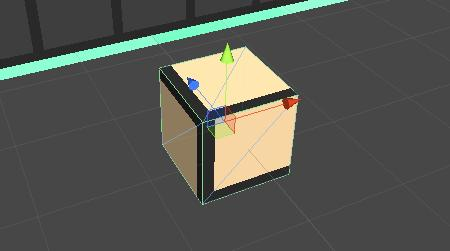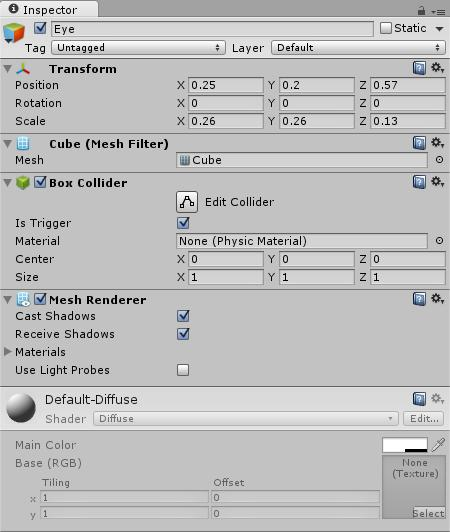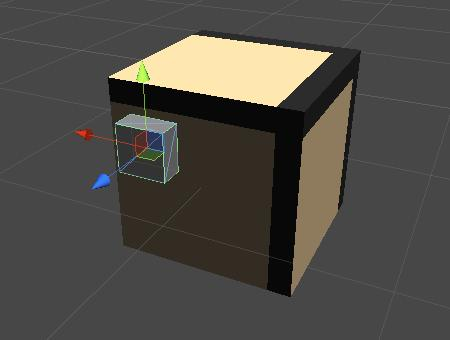-tex_eye.png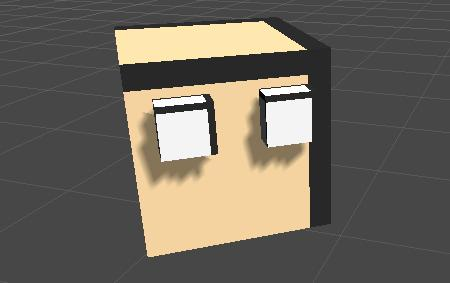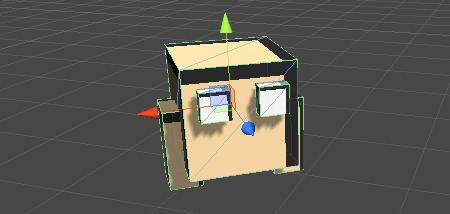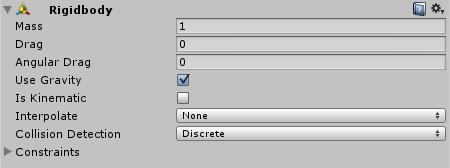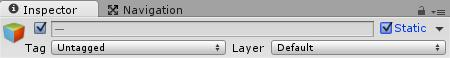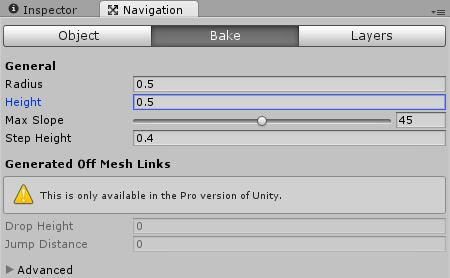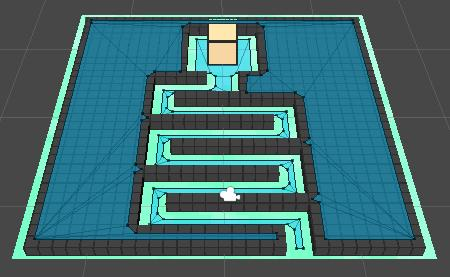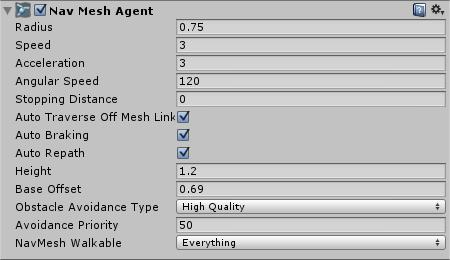[C#] 纯文本查看 复制代码

?

 `GetComponent.destination =``new` `Vector3(1, 2, 3);`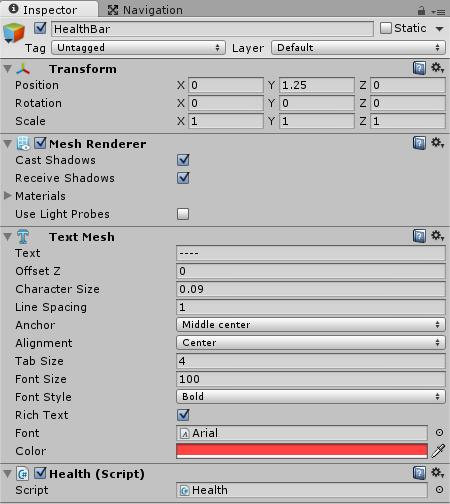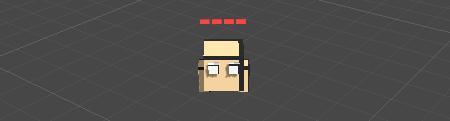[C#] 纯文本查看 复制代码

?

 12345678 `using` `UnityEngine;``using` `System.Collections;``public` `class` `Monster : MonoBehaviour {`` ``// Use this for initialization`` ``void` `Start () {`` ``}[/align][align=left]`

[C#] 纯文本查看 复制代码

?

 1234 ` ``// Update is called once per frame`` ``void` `Update () {`` ``}``}`

[C#] 纯文本查看 复制代码

?

 123456789 `using` `UnityEngine;``using` `System.Collections;``public` `class` `Monster : MonoBehaviour {`` ``// Use this for initialization`` ``void` `Start () {`` ``}``}`

[C#] 纯文本查看 复制代码

?

 123456 `// Use this for initialization``void` `Start () {`` ``// Navigate to Castle`` ``GameObject castle = GameObject.Find(``"Castle"``);`` ``if` `(castle)`` ``GetComponent().destination = castle.transform.position;``}`

[C#] 纯文本查看 复制代码

?

 12345 `void` `OnTriggerEnter(Collider co) {`` ``// If castle then deal Damage`` ``if` `(co.name == ``"Castle"``) {`` ``co.GetComponentInChildren().decrease();`` ``}``}`

[C#] 纯文本查看 复制代码

?

 123456 `void` `OnTriggerEnter(Collider co) {`` ``// If castle then deal Damage, destroy self`` ``if` `(co.name == ``"Castle"``) {`` ``co.GetComponentInChildren().decrease();`` ``Destroy(gameObject);`` ``}``}`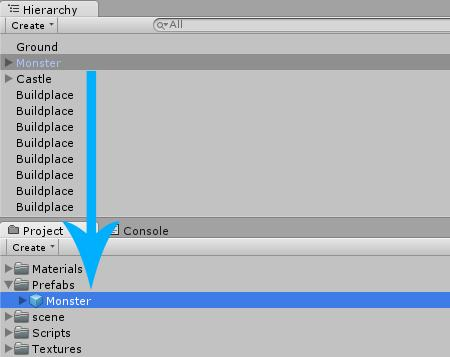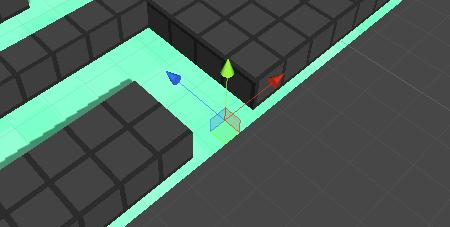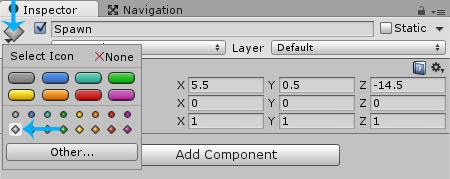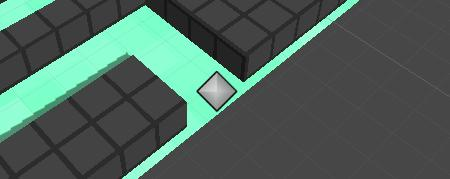[C#] 纯文本查看 复制代码

?

 0102030405060708091011121314 `using` `UnityEngine;``using` `System.Collections;``public` `class` `Spawn : MonoBehaviour {`` ``// Use this for initialization`` ``void` `Start () {`` ``}`` ``// Update is called once per frame`` ``void` `Update () {`` ``}``}`

[C#] 纯文本查看 复制代码

?

 123456789 `using` `UnityEngine;``using` `System.Collections;``public` `class` `Spawn : MonoBehaviour {`` ``// Use this for initialization`` ``void` `Start () {`` ``}``}`

[C#] 纯文本查看 复制代码

?

 0102030405060708091011 `using` `UnityEngine;``using` `System.Collections;``public` `class` `Spawn : MonoBehaviour {`` ``// The Monster that should be spawned`` ``public` `GameObject monsterPrefab;`` ``// Use this for initialization`` ``void` `Start () {`` ``}``}`

[C#] 纯文本查看 复制代码

?

 0102030405060708091011121314 `using` `UnityEngine;``using` `System.Collections;``public` `class` `Spawn : MonoBehaviour {`` ``// The Monster that should be spawned`` ``public` `GameObject monsterPrefab;`` ``// Spawn Delay in seconds`` ``public` `float` `interval = 3;`` ``// Use this for initialization`` ``void` `Start () {`` ``}``}`

[C#] 纯文本查看 复制代码

?

 010203040506070809101112131415161718 `using` `UnityEngine;``using` `System.Collections;``public` `class` `Spawn : MonoBehaviour {`` ``// The Monster that should be spawned`` ``public` `GameObject monsterPrefab;`` ``// Spawn Delay in seconds`` ``public` `float` `interval = 3;`` ``// Use this for initialization`` ``void` `Start() {`` ``InvokeRepeating(``"SpawnNext"``, interval, interval);`` ``}`` ``void` `SpawnNext() {`` ``Instantiate(monsterPrefab, transform.position, Quaternion.identity);`` ``}``}`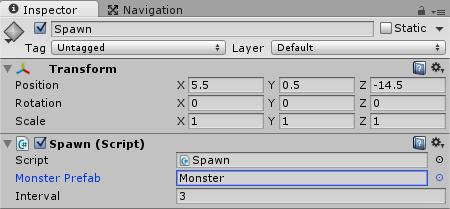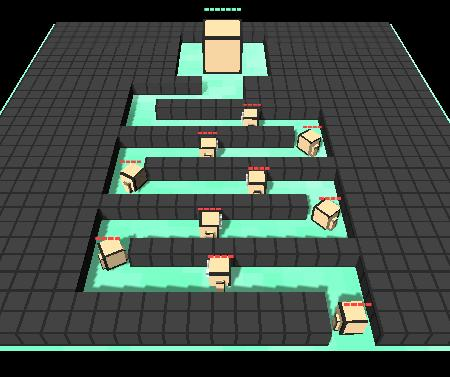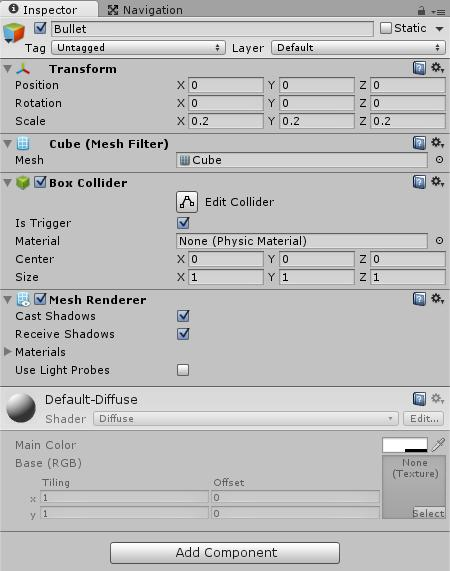tex_bullet.png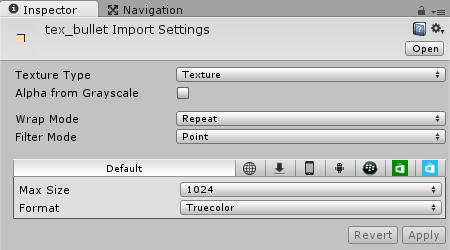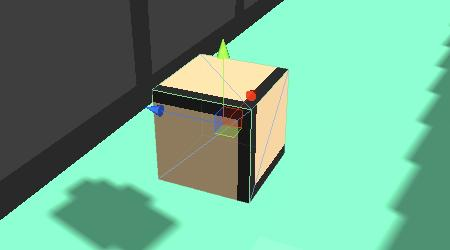[C#] 纯文本查看 复制代码

?

 0102030405060708091011121314 `using` `UnityEngine;``using` `System.Collections;``public` `class` `Bullet : MonoBehaviour {`` ``// Use this for initialization`` ``void` `Start () {`` ``}`` ``// Update is called once per frame`` ``void` `Update () {`` ``}``}`

[C#] 纯文本查看 复制代码

?

 12345678 `using` `UnityEngine;``using` `System.Collections;``public` `class` `Bullet : MonoBehaviour {`` ``void` `FixedUpdate() {`` ``}``}`

[C#] 纯文本查看 复制代码

?

 01020304050607080910111213 `using` `UnityEngine;``using` `System.Collections;``public` `class` `Bullet : MonoBehaviour {`` ``// Speed`` ``public` `float` `speed = 10;`` ``// Target (set by Tower)`` ``public` `Transform target; `` ``void` `FixedUpdate() {`` ``}``}`

[C#] 纯文本查看 复制代码

?

 1234 `void` `FixedUpdate() { `` ``// Fly towards the target `` ``Vector3 dir = target.position - transform.position;`` ``GetComponent().velocity = dir.normalized * speed;``}`

[C#] 纯文本查看 复制代码

?

 01020304050607080910 `void` `FixedUpdate() { `` ``// Still has a Target?`` ``if` `(target) {`` ``// Fly towards the target `` ``Vector3 dir = target.position - transform.position;`` ``GetComponent().velocity = dir.normalized * speed;`` ``} ``else` `{`` ``// Otherwise destroy self`` ``Destroy(gameObject);`` ``}``}`

[C#] 纯文本查看 复制代码

?

 123456 `void` `OnTriggerEnter(Collider co) {`` ``Health health = co.GetComponentInChildren();`` ``if` `(health) {`` ``health.decrease();`` ``Destroy(gameObject);`` ``}``}`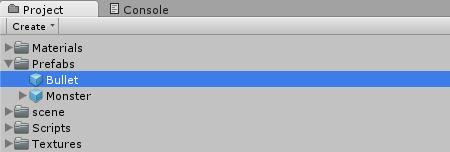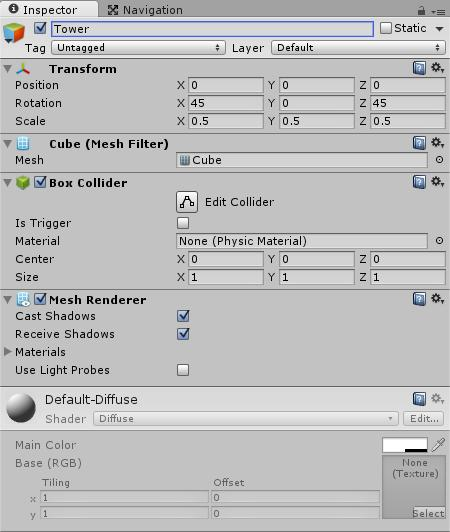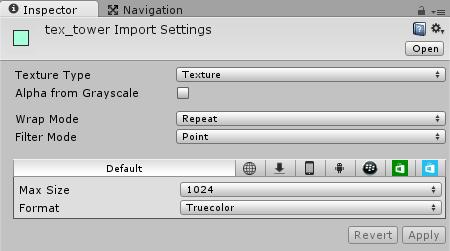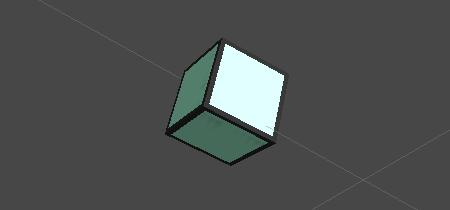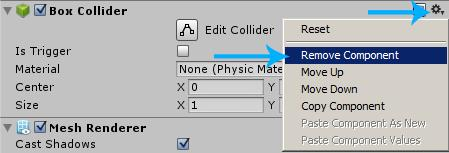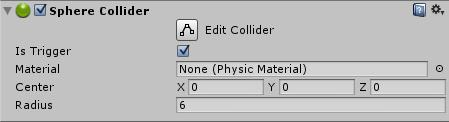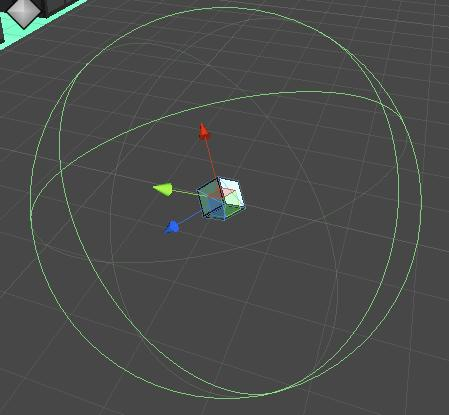[C#] 纯文本查看 复制代码

?

 0102030405060708091011121314 `using` `UnityEngine;``using` `System.Collections;``public` `class` `Tower : MonoBehaviour {`` ``// Use this for initialization`` ``void` `Start () {`` ``}`` ``// Update is called once per frame`` ``void` `Update () {`` ``}``}`

[C#] 纯文本查看 复制代码

?

 123456789 `using` `UnityEngine;``using` `System.Collections;``public` `class` `Tower : MonoBehaviour {`` ``// Update is called once per frame`` ``void` `Update () {`` ``}``}`

[C#] 纯文本查看 复制代码

?

 01020304050607080910111213 `using` `UnityEngine;``using` `System.Collections;``public` `class` `Tower : MonoBehaviour {`` ``// The Bullet`` ``public` `GameObject bulletPrefab;`` ``// Update is called once per frame[mw_shl_code=csharp,true]void OnTriggerEnter(Collider co) {`` ``// Was it a Monster? Then Shoot it`` ``if` `(co.GetComponent()) {`` ``GameObject g = (GameObject)Instantiate(bulletPrefab, transform.position, Quaternion.identity);`` ``g.GetComponent().target = co.transform;`` ``}``}`

void Update () {

}

}

[/mw_shl_code]

[C#] 纯文本查看 复制代码

?

 123456 `void` `OnTriggerEnter(Collider co) {`` ``// Was it a Monster? Then Shoot it`` ``if` `(co.GetComponent()) {`` ``GameObject g = (GameObject)Instantiate(bulletPrefab, transform.position, Quaternion.identity);`` ``g.GetComponent().target = co.transform;`` ``}``}`

[C#] 纯文本查看 复制代码

?

 010203040506070809101112131415161718192021 `using` `UnityEngine;``using` `System.Collections;``public` `class` `Tower : MonoBehaviour {`` ``// The Bullet`` ``public` `GameObject bulletPrefab;`` ``// Rotation Speed`` ``public` `float` `rotationSpeed = 35;`` ``void` `Update() {`` ``transform.Rotate(Vector3.up * Time.deltaTime * rotationSpeed, Space.World);`` ``}`` ``void` `OnTriggerEnter(Collider co) {`` ``// Was it a Monster? Then Shoot it`` ``if` `(co.GetComponent()) {`` ``GameObject g = (GameObject)Instantiate(bulletPrefab, transform.position, Quaternion.identity);`` ``g.GetComponent().target = co.transform;`` ``}`` ``}``}`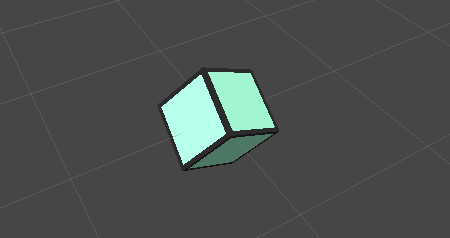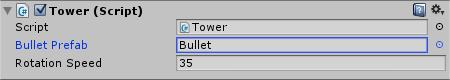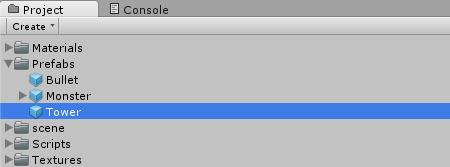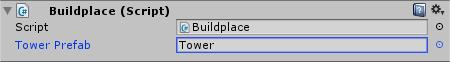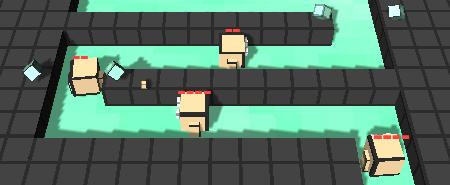Unity宝塔对抗教程的源码及项目的文件能够被下载。